# AP Physics C: Mechanics : Understanding Conservation of Angular Momentum

## Example Questions

### Example Question #1 : Understanding Conservation Of Angular Momentum

A mouse sits at the edge of a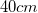diameter record, rotating on a turntable at fifteen revolutions per minute. If the mouse walks straight inwards to a point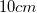from the center, what will be its new angular velocity? Assume the mass of the record is negligible.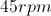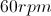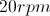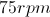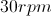Explanation:

Relevant equations: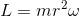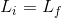Write an expression for the initial angular momentum.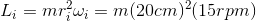Write an expression for the final angular momentum.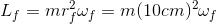Apply conservation of angular momentum, setting these two expressions equal to one another.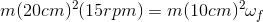Solve for the final angular velocity.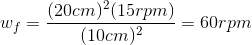### Example Question #71 : Motion

An ice skater begins to spin, starting with his arms spread out as far as possible, parallel to the ice. He pulls his arms into his body, and then raises them completely vertically towards the ceiling. As the skater pulls his arms inward, his angular velocity will __________, and as he raises his arms vertically his angular velocity will __________.

increase . . . decrease

decrease . . . increase

increase . . . stay the same

decrease . . . stay the same

increase . . . stay the same

Explanation:

Through conservation of angular momentum, as moment of inertia decreases, the angular velocity increases. Moment of inertia is dependent on the distribution of the spinning body's mass away from the center of mass—as the skater brings his arms in, he lowers his moment of inertia, thus increasing his angular velocity. If he raises his arms completely vertically from this point, it does not change the radius of mass distributions (from the center of his body), thus maintaining his angular velocity.

### Example Question #72 : Motion

Sarah spins a ball of massattached to a string of lengtharound her head with a velocity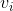. If the ball splits in half, losing exactly one-half of its mass instantaneously, what is its new velocity,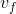?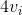(no change in velocity)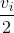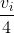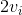Explanation:

By the conservation of angular momentum, the angular momentum, is equal to the product of the mass, angular velocity, and radius (or length of the rope in this case). The equation relating these terms is: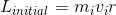Here,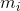is the initial mass,is the initial angular velocity, andis the length of the rope, which remains constant. Angular momentum must be conserved, thus: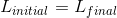Substitute.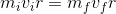We are given that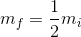andis the final velocity. Plug in and solve.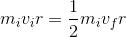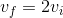### Example Question #73 : Motion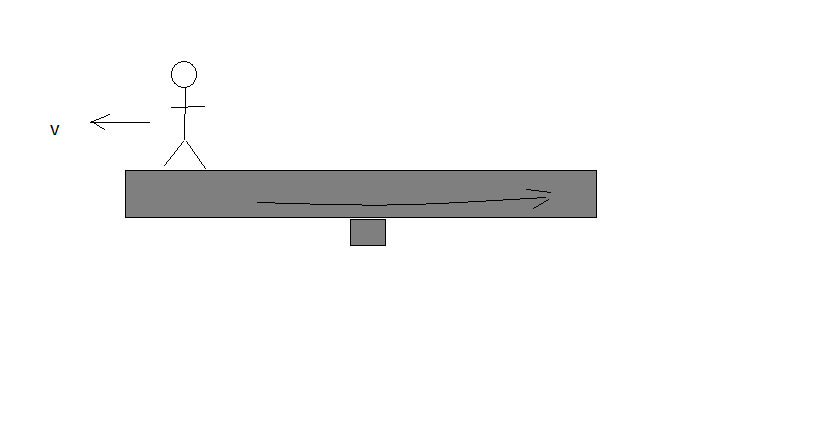A child is standing at the center of a frictionless, rotating platform. Both the child and the platform are rotating with and initial angular velocity,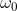. The child begins to walk slowly toward the edge of the platform. Which quantity will decrease as the child walks?

Total angular inertia

Total angular momentum

Total momentum

Rotational inertia of the disk

Total rotational kinetic energy

Since the platform is frictionless, as the child walks, angular momentum is conserved. However, since there is an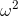term in the kinetic energy expression, as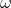decreased due to the increase of inertia, it affects the energy more, and it decreases. From the reference frame of the disk, the child feels an outward directed force, and thus is doing negative work. This is the same principle that allows ice skaters to increase their spinning speed.